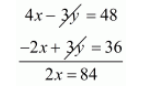# A wizard having powers of mystic in candations and magical medicines seeing a cock,`
Question:

A wizard having powers of mystic in candations and magical medicines seeing a cock, fight going on, spoke privately to both the owners of cocks. To one he said; if your bird wins, than you give me your stake-money, but if you do not win, I shall give you two third of that'. Going to the other, he promised in the same way to give three fourths. From both of them his gain would be only 12 gold coins. Find the the stake of money each of the cock-owners have.

Solution:

Let the strike money of first cock-owner be $R s . x$ and of second cock-owner be $R s . y$ respectively. Then we have,

$x-\frac{3}{4} y=12$

$\frac{4 x-3 y}{4}=12$

$4 x-3 y=12 \times 3$

$4 x-3 y=48 \cdots(i)$

For second cock-owner according to given condition we have,

$y-\frac{2}{3} x=12$

$\frac{3 y-2 x}{3}=12$

$3 y-2 x=12 \times 3$

$3 y-2 x=36 \cdots(i i)$

By subtracting $(i i)$ from $(i)$, we have,$x=\frac{84}{2}$

$x=42$

Putting $x=42$ in equation (ii) we get,

$3 y-2 x=36$

$3 y-2 \times 42=36$

$3 y-84=36$

$3 y=36+84$

$3 y=120$

$y=\frac{120}{3}$

$y=40$

Hence the stake of money first cock-owner is Rs. 42 and of second cock-owner is Rs. 40 respectively.